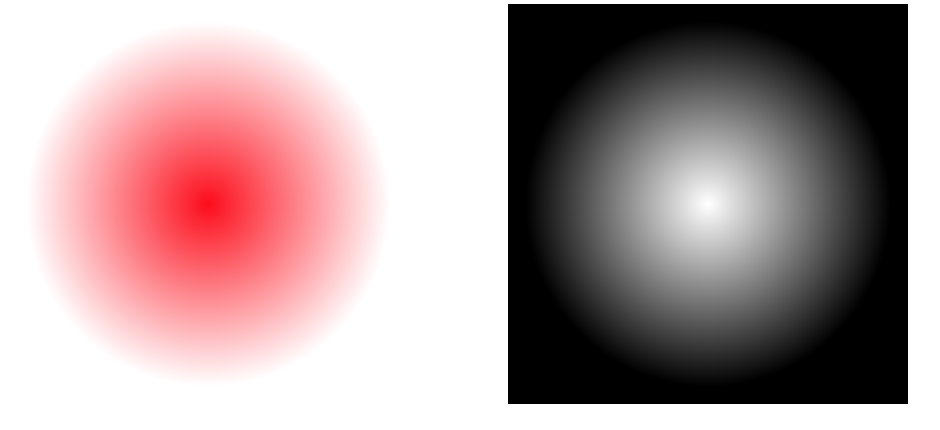#2019 iT 邦幫忙鐵人賽DAY 26
0
Modern Web

## clipping

``````<svg width="200" height="200">
<!-- 遮色片設定為寬100高200的矩形，位置(100,0) -->
<defs>
<clipPath id="cut">
<rect x="100" y="0" width="100" height="200" />
</clipPath>
</defs>
<!-- 被遮的圖案為半徑100的圓形，圓心位置(100,100) -->
<circle cx="100" cy="100" r="100" clip-path="url(#cut)" />
</svg>
````````````<svg width="500" height="500">
<defs>
<radialGradient id="a2" cx="50%" cy="50%" fx="50%" fy="50%" r="76%" >
<stop stop-color="#ffffff" offset="0%"></stop>
<stop stop-color="black" offset="60%"></stop>
<rect x="0" y="0" width="200" height="200" fill="url(#a2)"/>
</defs>
<rect x="0" y="0" width="200" height="200" fill="red" mask="url(#Mask)" />
<rect x="250" y="0" width="200" height="200" fill="url(#a2)"  />
</svg>
``````[補充]透明度的不同，也可以做不同的遮罩效果

(這裡有有趣的遮罩範例來看看)

～SVG龜速學習中，如有疑問或是錯誤，歡迎不吝指教～